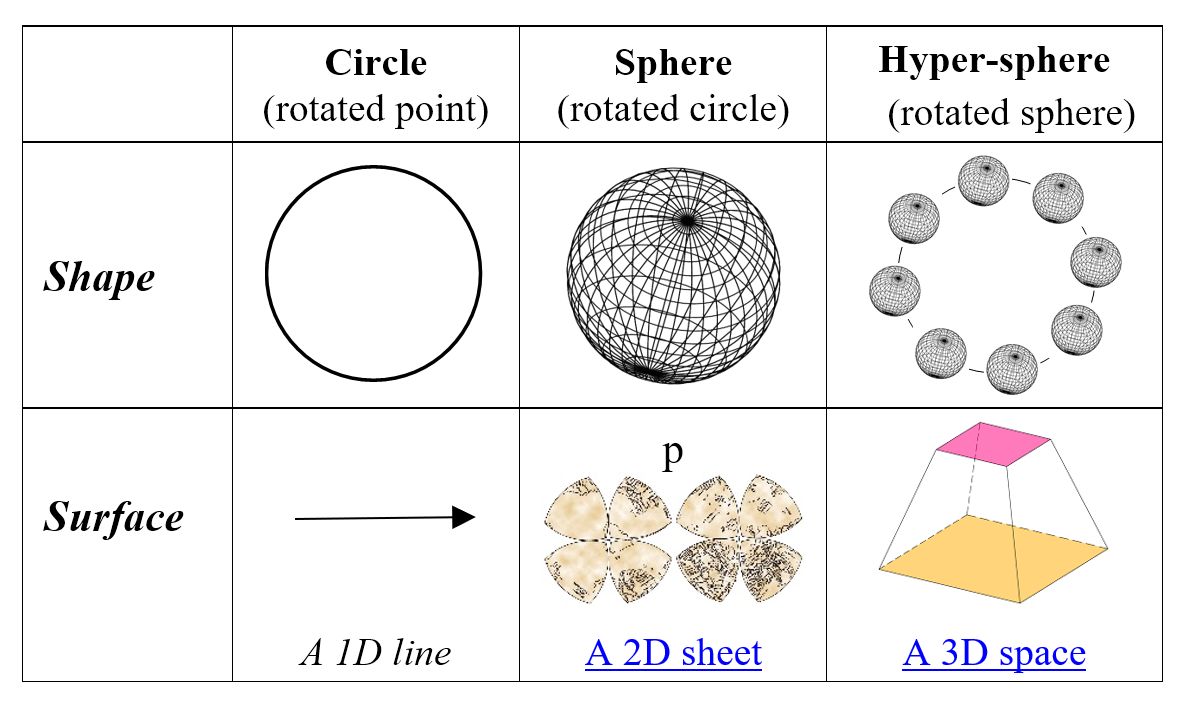# QR2.2.5 A Polar Space

Cartesian space is so deeply ingrained in western thought that one might think it is the only way to describe a space but it isn’t so. Euclid derived Cartesian coordinates by extending a point to a line, extending the line to a plane and then extending the plane to a volume. But instead of using straight lines, one can extend a point using rotations to derive polar coordinates.

Given a network, one can rotate a point to create a circle and then repeat. The first circle defines one-dimension, as every node has two neighbors giving left and right directions and the distance between two points is the number of link connections (Figure 2.3). The network architecture, or how the nodes connect, defines distance and direction as a node directly linked to another is “near” while another many links away is “far”. The advantage of a circular dimension is that it is finite not potentially infinite and it does not have its zero point on itself.

So just as Euclid did for a line, the circle in Figure 2.3 can be rotated at right angles to give a two-dimensional sphere (Figure 2.4).

A “Flatlander” confined to this surface would see a space that is:

1. Finite. Has finite number of points.

2. Unbounded. Moving in any direction never ends.

3. Has no center. No point on the sphere surface is the center.

4. Approximately flat. If the sphere is large enough.

5. Simply connected. A mathematical term that means any loop on it can shrink to a point.

In other words, this surface performs just like our space but with only two dimensions.

Now just as rotating a circle gives a sphere with a two-dimensional surface, so rotating a sphere gives a hypersphere with a surface of three dimensions (Figure 2.5).Figure 2.5 A Hypersphere has a three dimensional surface

A hypersphere is what you get by rotating a sphere just as a sphere is what you get by rotating a circle and in mathematics, a hypersphere surface has three dimensions just as a sphere surface has two. Centuries earlier, the mathematician Riemann speculated that our space was a hypersphere surface because such a surface is unbounded, simply connected and three-dimensional just as our space is. The logic is even more convincing today, when Einstein argues that space curves like a surface and cosmology says it expands everywhere at once like a balloon surface. Logically, our 3D space could easily be a surface in a four-dimensional bulk:

When it comes to the visible universe the situation could be subtle. The three-dimensional volume of space might be the surface area of a four dimensional volume(Barrow, 2007) p180

Davies makes the case even more clearly:

“… the shape of space resembles a three-dimensional version of the surface of a sphere, which is called a hypersphere.(P. Davies, 2006) p45

If our space is a hypersphere surface within a four-dimensional quantum network, why then does space appear flat not curved? The simple answer is that the surface of a hypersphere bubble that has expanded for over 14 billion years would seem flat to us.

Next